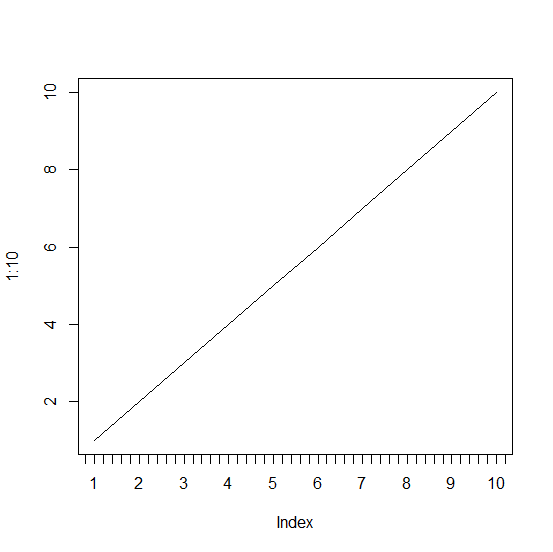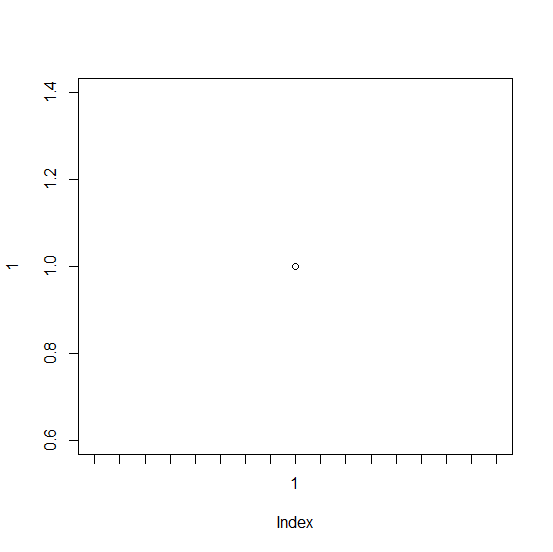# How to create a plot with tick marks between X-axis labels in base R?

To create a plot with tick marks manually between X-axis values in base R, we first need to create the plot without X-axis labels then add the axis values using axis function with appropriate labels, this will create tick marks as well as labels. After this step, we would need to use the axis function again to add the tick marks without labels.

## Example

Live Demo

plot(1:10,xaxt='n',type="l")
axis(1,at=1:10)
axis(1,at=seq(0,11,0.2),labels=NA)

## Output## Example

Live Demo

plot(1,xaxt='n')
axis(1,at=1)
axis(1,at=seq(0,2,0.05),labels=NA)

## Output